1. /
2. CBSE
3. /
4. Class 11
5. /
6. Introduction to Index Number...

# Introduction to Index Number class 11 Notes Economics

CBSE Economics Chapter 8 Introduction to Index Number class 11 Notes Economics in PDF are available for free download in myCBSEguide mobile app. The best app for CBSE students now provides Introduction to Index Number class 11 Notes Economics latest chapter wise notes for quick preparation of CBSE exams and school based annual examinations. Class 11 Economics notes on Chapter 8 Introduction to Index Number class 11 Notes Economics are also available for download in CBSE Guide website.

## CBSE Guide Introduction to Index Number class 11 Notes

CBSE guide notes are the comprehensive notes which covers the latest syllabus of CBSE and NCERT. It includes all the topics given in NCERT class 11 Economics text book. Users can download CBSE guide quick revision notes from myCBSEguide mobile app and my CBSE guide website.

## Introduction to Index Number class 11 Notes Economics

Download CBSE class 11th revision notes for Chapter 8 Introduction to Index Number class 11 Notes Economics in PDF format for free. Download revision notes for Introduction to Index Number class 11 Notes Economics and score high in exams. These are the Introduction to Index Number class 11 Notes Economics prepared by team of expert teachers. The revision notes help you revise the whole chapter in minutes. Revising notes in exam days is on of the best tips recommended by teachers during exam days.

CBSE Class 11 Economics
Revision Notes
Chapter – 8
Introduction to Index Number class 11 Notes Economics

Introduction to index number: An index number is a statistical device for measuring changes in the magnitude of a group of related variables.

Features of Index Number

1. Index numbers are expressed in terms of percentages. However, percentage sign (%) is never used.
2. Index numbers are relative measurement of group of data.
3. Index numbers offer a precise measurement of the quantitative change in the concerned variables over time.
4. Index number show changes in terms of averages.
5. They are expressed in numbers.
6. Index number facilitates the comparative study over different time period.

Importance of Index number

1. It serves as a barometer for measuring the value of money.
2. Gives knowledge about change in standard of living.
3. It helps the business community in planning their decision.

Limitation of Index Number

1. Limited applicability
2. International comparison is not possible
3. Limited coverage
4. Difficulty in the construction of index number

Types of Index numbers:
(i) Wholesale price index (WPI)
(ii) Consumer price index (CPI) or Cost of living index
(iii) Index of industrial production (IIP)
(iv) Index of Agricultural production (IAP)
(v) Sensex

Methods of constructing index numbers:
1. Construction of Simple Index numbers
a. Simple Aggregative Method
b. Simple Average of Price Relatives Method
2. Construction of weighted Index numbers
a. weighted Average of Price Relative Method
b. Weighted Aggregative Method
Simple aggregative method: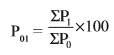Here,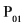= Price index of the current year.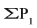= Sum of the prices of the commodities in the current year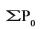= Sum of the prices of the commodities in the base year

Current year: Current year is the year for which average change is to be measured or index of index number is to be calculated.
Base year: Base year is the year of reference from which we want measure extent of change in the current year. The index number of base year is generally assumed to be 100.

Simple average of price Relatives method: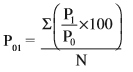Here,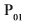= Price index of the current year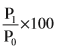= Price relatives
N = Number of commodities

Weighted average of price relative method: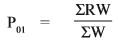Here,= Index number for the current year in relation to base year
W = Weight, R = Price relatives i.e.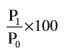Weighted Aggregative method:
(i) Laspeyre’s method  :- P01 =
(ii) Pasche’s method :- P01 =
(iii) Fisher’s Method :- P01 =

Some Important index numbers:
(i) Consumer price index (CPI)
: CPI is also known as the cost of living index, measures the average change in retail prices.
Methods of constructing CPI:
Here,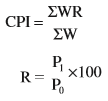W = Weights

(B) Aggregative expenditure method: P01 =
(ii) Wholesale price index (WPI): WPI Indicates the change in the general price level.
(iii) Index of industrial production (IIP): IIP is used to measure the relative increase or decrease in the level of industrial production.
IIP=
Here,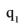= Level of Production in the current year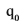= Level of production in the base year
W = Weight
(iv) Index of agriculture production (IAP)
IAP is used to study the rise and fall of the yield of principle crops from one period to other period.
(v) Sensex: Sensex is the short form of Bombay stock exchange sensitive index with 1978-79 as base. It is the benchmark index for the Indian stock market.
It consists or 30 stocks which represent 13 sectors of the economy and the companies are the leaders in their respective industries.

Problems in construction of index numbers:
(i) Purpose of index number.
(ii) Selection of base year.
(iii) Selection of items.
(iv) Selection of the prices of items.
(v) Selection of method of weighting
(vi) Selection of sources of data
(vii) Choice of an average.
(viii) Choice of method.

Uses of index numbers:
(i) To measure the purchasing power of money.
(ii) Knowledge of change in standard of living.
(iii) Adjustment in salaries and allowances.
(iv) Help in framing suitable policies.
(v) As economic barometers.

Inflation and index numbers:
Inflation is described a situation characterised by a sustained increase in the general price level. Generally, inflation is measured in terms of wholesale price index.
Rate of inflation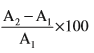Here,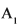= WPI for week first (1)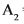= WPI for week second (2)

## Introduction to Index Number class 11 Notes

• CBSE Revision notes for Class 11 Economics PDF
• CBSE Revision notes Class 11 Economics – CBSE
• CBSE Revisions notes and Key Points Class 11 Economics
• Summary of the NCERT books all chapters in Economics class 11
• Short notes for CBSE class 11th Economics
• Key notes and chapter summary of Economics class 11
• Quick revision notes for CBSE exams

## CBSE Class-11 Revision Notes and Key Points

Introduction to Index Number class 11 Notes Economics. CBSE quick revision note for class-11 Mathematics, Physics, Chemistry, Biology and other subject are very helpful to revise the whole syllabus during exam days. The revision notes covers all important formulas and concepts given in the chapter. Even if you wish to have an overview of a chapter, quick revision notes are here to do if for you. These notes will certainly save your time during stressful exam days.

To download Introduction to Index Number class 11 Notes, sample paper for class 11 Chemistry, Physics, Biology, History, Political Science, Economics, Geography, Computer Science, Home Science, Accountancy, Business Studies and Home Science; do check myCBSEguide app or website. myCBSEguide provides sample papers with solution, test papers for chapter-wise practice, NCERT solutions, NCERT Exemplar solutions, quick revision notes for ready reference, CBSE guess papers and CBSE important question papers. Sample Paper all are made available through the best app for CBSE students and myCBSEguide website.Test Generator

Create question paper PDF and online tests with your own name & logo in minutes.myCBSEguide

Question Bank, Mock Tests, Exam Papers, NCERT Solutions, Sample Papers, Notes